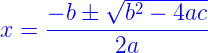# Equation Formula

Quadratic equation is one of the fundamental concept of algebra. By using formula, this quadratic form can be solved and solutions can be verified too. In this case there are two solutions. The formula for equation is given below.
Let ax2+ bx + c = 0 is the quadratic equation, the solution will be,## Equation Problems

Let us discuss the solutions of quadratic equations.

### Solved Examples

Question 1: Find out the roots of the given equation x2 + 2x – 3 = 0.

Solution:

x2 + 2x – 3 = 0
Here a = 1, b = 2 and c = -3
The formula for the solution,

x =

$$\begin{array}{l}\frac{-b \pm \sqrt{b^{2}-4ac}}{2a}\end{array}$$

x =

$$\begin{array}{l}\frac{-2 \pm \sqrt{2^{2}-4\times1\times-3}}{2\times1}\end{array}$$

x =

$$\begin{array}{l}\frac{-2 \pm \sqrt{4+12}}{2}\end{array}$$

x =

$$\begin{array}{l}\frac{-2+4}{2}\end{array}$$
= 1 or x =
$$\begin{array}{l}\frac{-2-4}{2}\end{array}$$
= -3

Question 2: Find out the roots of 2x2 – 3x – 5 = 0.

Solution:

2x2 – 3x – 5 = 0
Here a = 2, b = -3 and c = -5
The formula for the solution,

x =

$$\begin{array}{l}\frac{-b \pm \sqrt{b^{2}-4ac}}{2a}\end{array}$$

x =
$$\begin{array}{l}\frac{3\pm \sqrt{3^{2}-4\times2\times-5}}{2\times2}\end{array}$$

x =
$$\begin{array}{l}\frac{3\pm \sqrt{49}}{4}\end{array}$$

x =

$$\begin{array}{l}\frac{3+7}{4}\end{array}$$
=
$$\begin{array}{l}\frac{5}{2}\end{array}$$
or x =
$$\begin{array}{l}\frac{3-7}{4}\end{array}$$
= -1

 More topics in Equation Formula Linear Equations Formula